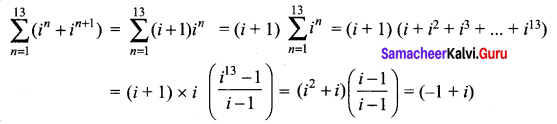Samacheer Kalvi 12th Maths Solutions Chapter 2 Complex Numbers Ex 2.1

Tamilnadu Samacheer Kalvi 12th Maths Solutions Chapter 2 Complex Numbers Ex 2.1

Simplify the following:

Question 1.
i1947 + i1950
Solution: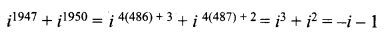Question 2.
i1948 – i-1869
Solution: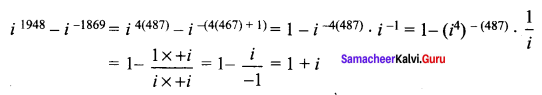Question3.
$$\sum_{n=1}^{12} i^{n}$$
Solution: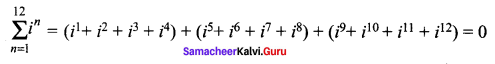Question 4.
$$i^{59}+\frac{1}{i^{59}}$$
Solution: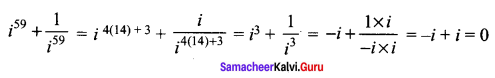Question 5.
i i2 i3 …….. i2000
Solution: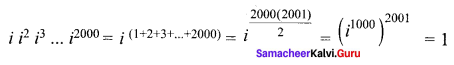Question 6.
$$\sum_{n=1}^{10} i^{n+50}$$
Solution: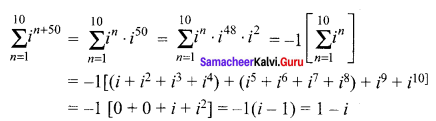Samacheer Kalvi 12th Maths Solutions Chapter 3 Theory of Equations Ex 2.1 Additional Problems

Question 1.
Evaluate the following: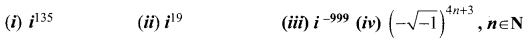Solution:
135 leaves remainder as 3 when it is divided by 4.
∴ i135 = i3 = -i

(ii) The remainder is 3 when 19 is divided by 4.
∴ i19 = i3 = -i

(iii) We have, i999 = 1/i999
On dividing 999 by 4, we obtain 3 as the remainder.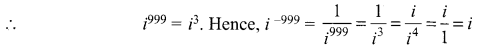(iv)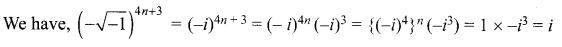Question 2.
Show that: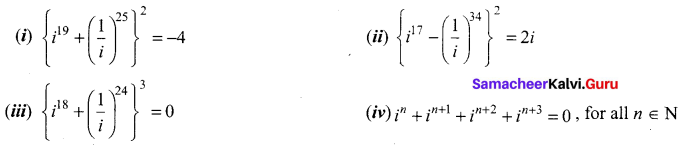Solution: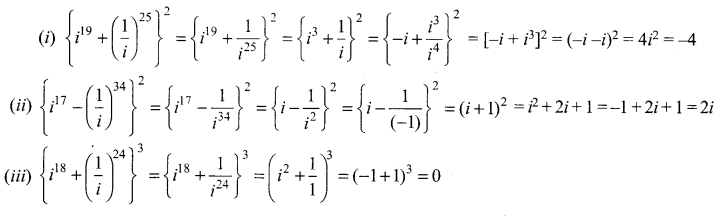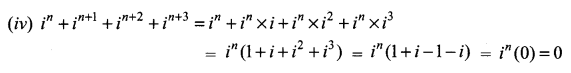Question 3.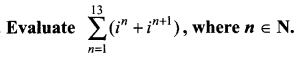Solution: